# How to VLOOKUP with Dropdown List in Excel?

We often use VLOOKUP function to find proper value by certain conditions in excel. And we often create a dropdown list to filter value in excel. If you combine these two features together, it can help us solve a lot of search problems by some conditions, and it will bring us a lot of convenience. For example, we have two columns of data, one column lists the names and another one lists the sales, we want to choose a value from the Name dropdown list, and then show the corresponding sales value in the adjacent cell will, in this case we need to use VLOOKUP function. In this article we will use above case to illustrate how can we use the combination of  VLOOKUP and dropdown list.

We have a table of Name and Sales, see below screenshot: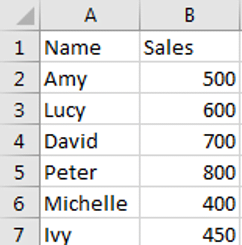And in column E, create a dropdown list of Names, when you select a name from dropdown list, the relative sales will be displayed in adjacent cell. See example below: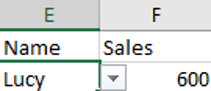So, if we want implement this feature, we need to use VLOOKUP function in F2 in this case. Please see steps below.

## Combine VLOOKUP and Dropdown List in Excel

Step 1: Create a dropdown list for Name column. In E2, click Data->Data Validation to load Data Validation window.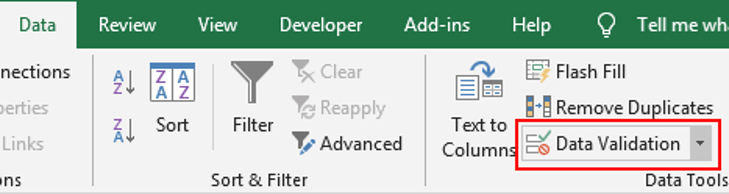Step 2: In Data Validation window, enter Settings tab, select List under Arrow field, enter =\$A\$2:\$A\$7 in Source field, then click OK.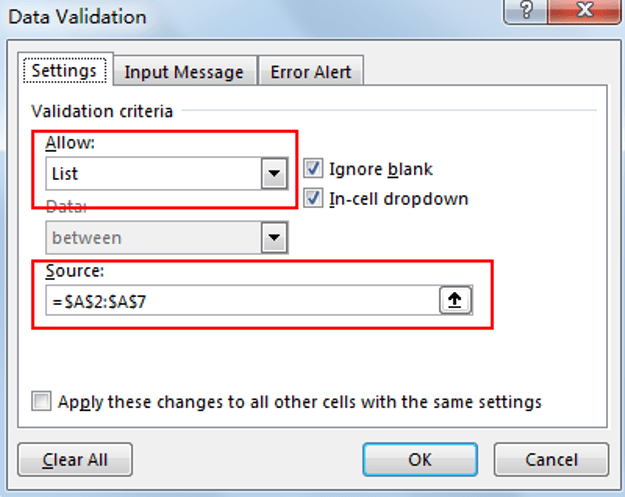Step 3: Verify that Dropdown list is created properly.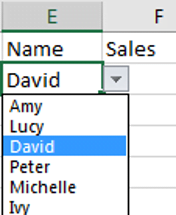Step 4: In F2 enter the formula =VLOOKUP(E2,\$A\$2:\$B\$7,2,FALSE).

In above formula, E2 is the value we filtered from the dropdown list; \$A\$2:\$B\$7 is the absolute range for search E2.

Step 5: In E2 select a name from dropdown list to verify if above formula works normally.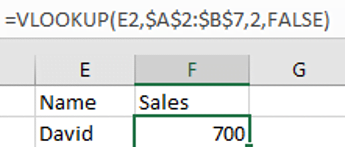From the test result we can find that it takes effective for cell F2.

### Related Functions

• Excel VLOOKUP function
The Excel VLOOKUP function lookup a value in the first column of the table and return the value in the same row based on index_num position.The syntax of the VLOOKUP function is as below:= VLOOKUP (lookup_value, table_array, column_index_num,[range_lookup])….